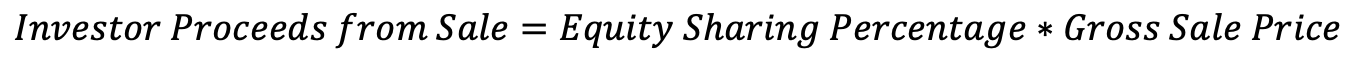DFW Properties Foreclosure Property Home Equity Structure
Share
Explore
Equity Exchange Program

#Equity Sharing

> Basics >
Equity Sharing Percentage
Definition
Equity sharing is one of the two main features of home investments through Vessel. When we refer to “buying" or "sharing" equity of a property, what we really mean is buying contractual rights to a percentage of the eventual gross sale price of the property. The initial Equity Sharing Percentage is determined as such:For example, a $100k investment into a property appraised at$400k would produce an initial Equity Sharing Percentage of 25%.
Demonstration
Try it out for yourself by adjusting the sliders below to see how the equity sharing percentage is calculated:
Appraised Home Value
$0000000 400000 Investment Amount$
000000
100000
Equity Sharing Percentage:
=
100000
/
400000
=
25
%
Proceeds from Sale
Definition
To calculate the amount the investors are owed at the time of sale (or ), multiply the equity sharing percentage and the gross sale price of the property. Closing costs are paid entirely by the property owner.Demonstration
If the property, originally appraised at
400000
, sells for:
$0000000 500000 then, given the 25 % Equity Sharing Percentage assumed in the previous section, the investor is entitled to: = 25 % x$
500000
= \$
125000
at the time of the property's sale.
Notes:
The Equity Sharing Percentage can increase during the life of the investment through at the property owner's discretions

This is not an actual purchase of equity; however, it uses to simulate something similar.

If this amount is greater than the sale price minus the balance due to any mortgage lenders in first position, then this amount would be the balance remaining after all mortgage payments are made. This is unlikely, because we only work with properties that are free & clear or have low mortgage balances.

Next topic: .